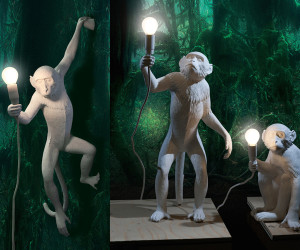# Monkey and Lamp Puzzle

1,124.8K Views

A very big building in which thousand monkeys are living is lighted by thousand lamps. Every lamp is connected to a unique on/off switch, which are numbered from 1 to 1000. At some moment, all lamps are switched off. But because it is becoming darker, the monkeys would like to switch on the lights. They will do this in the following way.

Monkey 1 presses all switches that are a multiple of 1.
Monkey 2 presses all switches that are a multiple of 2.
Monkey 3 presses all switches that are a multiple of 3.
Monkey 4 presses all switches that are a multiple of 4.
Etc., etc.

How many lamps are switched on after monkey 1000 pressed his switches?
And
which lamps are switched on?Only the lights connected to switches with a number that has an odd number of factors are left on.  These are the square numbers.  The largest square square number less than 1000 is 31^2 = 961, so there are 31 lights on connected to the switches 1^2, 2^2, … 31^2.

/*
Yep Thats Perfect!
Following is a java program to do the same thing.
*/
class k1{
public static int[] factorize (int a[], int p)
{
int count=0,no=p;
int b[]= new int[a.length];
b=a;
for(int i=2;i<=p;i++)
if(p%i==0){b[count++]=i;}
b[count++]=1;
return b;
}

public static int no_of_factors(int b[])
{
int counter=0;
for(int i=0;i<b.length-1;i++)
{
if(b[i+1]!=b[i]&&b[i]!=0) counter++;
}
return counter;
}

public static void main(String args[])
{
int on_count=0;
int a[]= new int;
for(int i=1;i<=1000;i++)
{
factorize(a,i);
int x = no_of_factors(a);
if(x%2!=0) on_count++;
for(int j=0;j<50;j++)a[j]=0;
}

System.out.print(on_count);

}// end of main
}// end of class